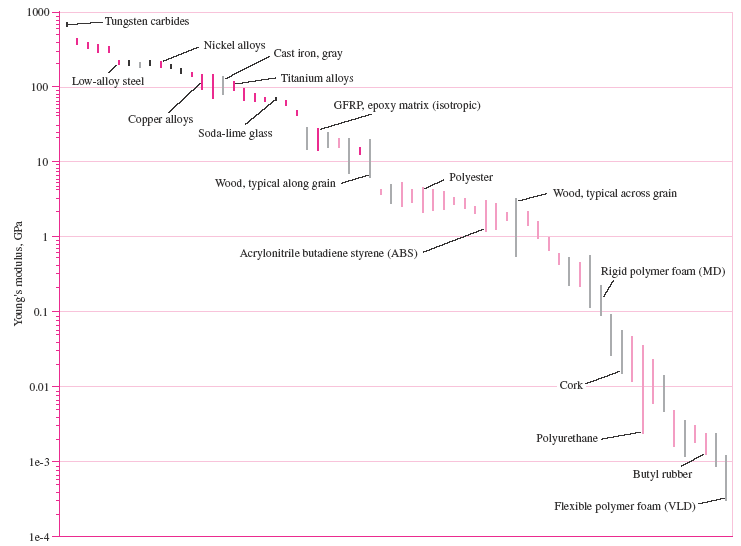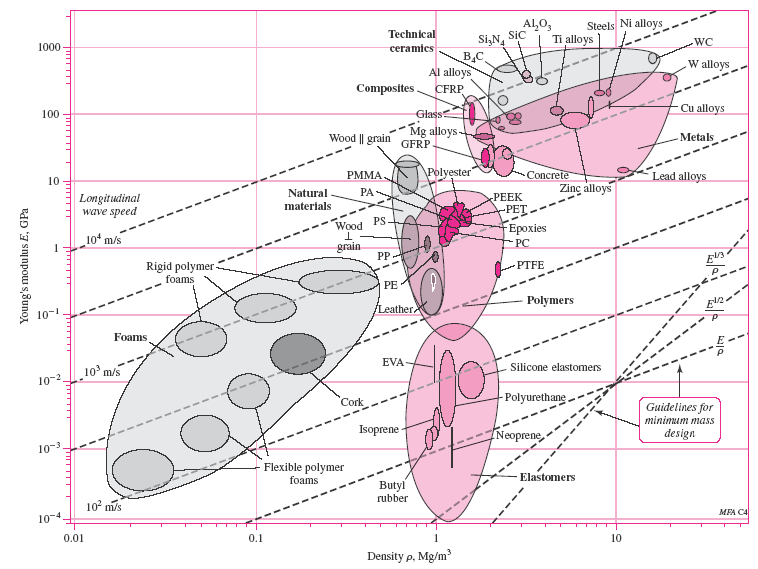### Young's Modulus, Modulus of Elasticity, Shear Modulus, Poisson's Ratio, Density

Modulus of Elasticity also know as Young's Modulus is the mathematical description of an object or substance's tendency to be deformed elastically (i.e., non-permanently) when a force is applied to it. The elastic modulus of an object is defined as the slope of its stress-strain curve in the elastic deformation region:

Eq. 1
λ = stress / strain

Where:

λ (lambda) = Elastic Modulus
stress = Restoring force caused due to the deformation divided by the area to which the force is applied
strain = Ratio of the change caused by the stress to the original state of the object

 Typical Values Modulus of Elasticity(Young's Modulus) Material Modulus of Elasticity, E (lb/in2 x 106) Shear Modulus of Elasticity, G  (lb/in2 x 106) Poisson's Ratio u Weight Density (lb/in3) Aluminum Alloys 10.2 3.9 0.33 0.098 Beryllium Copper 18.0 7.0 0.29 0.30 Carbon Steel 29.0 11.5 0.29 0.28 Cast Iron 14.5 6.0 0.21 0.26 Inconel 31.0 11.5 0.29 0.31 Magnesium 6.5 2.4 0.35 0.07 Molybdenum 48.0 17.1 0.31 0.37 Monel Metal 26.0 9.5 0.32 0.32 Nickel Silver 18.5 7.0 0.32 0.32 Nickel Steel 29.0 11.0 0.29 0.28 Nylon 1.5 0.6 - 0.04 Phosphor Bronze 16.1 6.0 0.35 0.30 Stainless Steel 27.6 10.6 0.31 0.28 Titanium 16.5 6.5 - 0.16Figure 1 Young’s modulus E for various materials. (Figure courtesy of Prof. Mike Ashby, Granta Design, Cambridge, U.K.)Figure 2 Young’s modulus E versus density ρ for various materials. (Figure courtesy of Prof. Mike Ashby, Granta Design, Cambridge, U.K.)

Related: